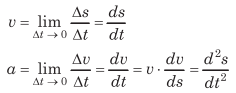# Uniformly Accelerated Motion | Definition, Equations – Motion in a Straight Line

Equations of Uniformly Accelerated Motion:
If a body starts with velocity (u) and after time t its velocity changes to v, if the uniform acceleration is a and the distance travelled in time t is s, then the following relations are obtained, which are called equations of uniformly accelerated motion.

We are giving a detailed and clear sheet on all Physics Notes that are very useful to understand the Basic Physics Concepts.

## Uniformly Accelerated Motion | Definition, Equations – Motion in a Straight Line

Uniformly Accelerated Motion Equations:
(i) v = u + at
(ii) s = ut + $$\frac{1}{2}$$ at²
(iii) v² = u² + 2as
(iv) Distance travelled in rath second.
sn = u + $$\frac{a}{2}$$ (2n -1)

If a body moves with uniform acceleration and velocity changes from u to v in a time interval, then the velocity at the mid-point of its path
= $$\frac{\sqrt{u^{2}+v^{2}}}{2}$$

Non-Uniformly Accelerated Motion Definition:
When acceleration of particle is not constant then motion is called non-uniformlly accelerated motion.
For one dimensional motion,where, Δs is displacement in time Δt, Δv is velocity in time Δt and a is instantaneous acceleration.
In component form,
$$\mathbf{a}=a_{x} \cdot \hat{\mathbf{i}}+a_{y} \cdot \hat{\mathbf{j}}+a_{z} \cdot \hat{\mathbf{k}}$$

where, ax = $$\frac{d v_{x}}{d t}$$, ay = $$\frac{d v_{y}}{d t}$$ and az = $$\frac{d v_{z}}{d t}$$

Motion in a Straight Line Topics: